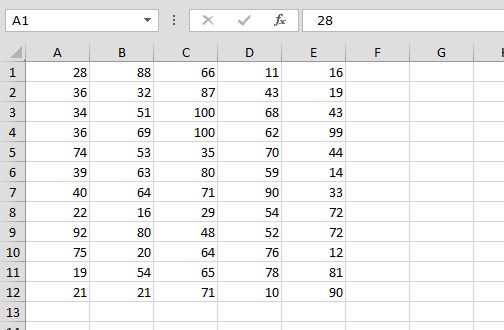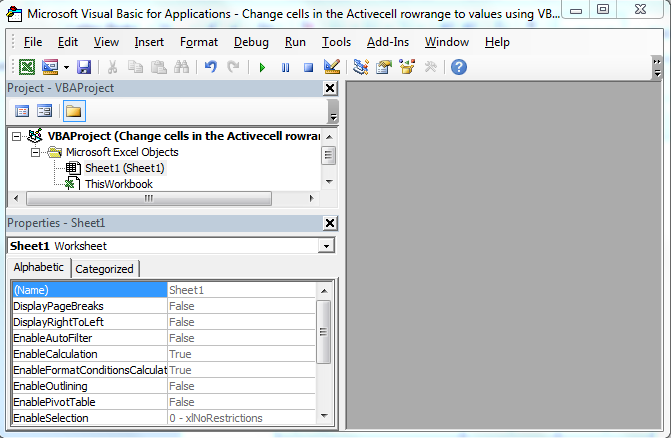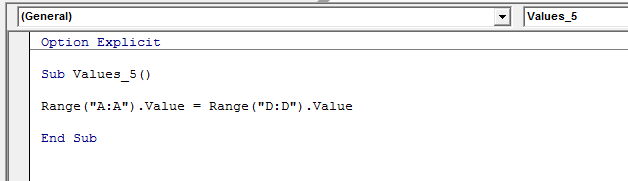Change cells in the Activecell rowrange to values using VBA in Microsoft Excel

In this chapter you will learn how to change cells in the active cell row range to values using VBA in Microsoft Excel.

Let’s take an example and understand how we write the VBA code for change the cells in the active cell row range to values.

How to change cell in the active cell row range to values?

We have data in Excel, in which we want the replace the active cell row range to values.• Press Alt+F11 key to open the Visual Basic Application• In VBA Project Double click on Sheet 1
• Enter the below given VBA CodeOption Explicit

Sub Values_5()

Range("A:A").Value = Range("D:D").Value

End Sub

To run the code press F5 key

• Define range value will get updated